# Analytic geometry

(diff) ← Older revision | Latest revision (diff) | Newer revision → (diff)

A branch of geometry. The fundamental concepts of analytic geometry are the simplest geometric elements (points, straight lines, planes, second-order curves and surfaces). The principal means of study in analytic geometry are the method of coordinates and the methods of elementary algebra. The genesis of the method of coordinates is closely linked with the intense development of astronomy, mechanics and technology in the 17th century. In his Géometrie, R. Descartes (1637) gave a clear and exhaustive account of this method and of the foundations of analytic geometry. P. Fermat, a contemporary of Descartes, was also familiar with the principles of this method. Subsequent development of analytic geometry is due to the studies of G. Leibniz, I. Newton and, particularly, L. Euler. The tools of analytic geometry were utilized by J.L. Lagrange in his construction of analytic mechanics and by G. Monge in differential geometry. In our own days, analytic geometry has no significance as an independent branch of science, but its methods are extensively employed in various fields of mathematics, mechanics, physics and other sciences.

The principle of the method of coordinates is as follows. Consider, for example, two mutually-perpendicular straight lines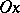andin a plane. These lines, including their direction, the coordinate origin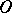and the selected scale unit, form a so-called Cartesian orthogonal system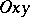of coordinates on the plane. The straight linesand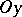are called the abscissa (axis) and the ordinate (axis) respectively. The location of any pointin the plane relative to this systemcan be determined as follows. Let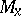and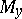be the projections ofontoand, and let the numbersand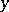be the magnitudes of the segments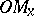and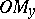(the magnitudeof the segment, for example, is equal to the length of this segment, taken with the plus sign if the direction fromtois the same as the direction of the straight line, and taken with the minus sign in the opposite case). The numbers(the abscissa) and(the ordinate) are said to be the Cartesian orthogonal coordinates of the pointin the system. A pointwith abscissaand ordinateis denoted by the symbol. The coordinates of a pointclearly determine its location with respect to the system.

Let a linebe given on a planewith a given Cartesian orthogonal coordinate system. Using the concept of coordinates of a point it is possible to introduce the concept of the equation of the linewith respect to systemas an equation of the type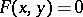, which will be satisfied by the coordinatesandof any pointon, but not by those of any point which does not lie on.

The basic idea of the method of coordinates on a plane is that the geometric properties of the line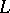can be clarified by studying its equationby analytic and algebraic tools. Thus, for instance, the problem of the number of intersection points between a straight line and a circle is reduced to the analytic problem of the number of solutions of the set of equations of the line and of the circle.

This was in fact the method of study of the properties of an ellipse, a hyperbola and a parabola, which are the lines of intersection between a circular cone and planes which do not pass through its vertex (cf. Conic sections).

The so-called algebraic curves of the first and second orders are a subject of systematic study in plane analytic geometry; in Cartesian orthogonal coordinates these are described by algebraic equations of the first and second degree, respectively. The curves of the first order are rectilinear and, conversely, any straight line is described by an algebraic equation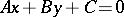of the first degree. The curves of the second order are described by equations of the form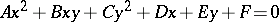. The principal technique in the study and classification of such curves is to select a Cartesian orthogonal coordinate system in which the equation assumes its simplest form, after which the simple equation is studied. Cf. Second-order curve.

In the analytic geometry of space, the Cartesian orthogonal coordinates areand(the abscissa, the ordinate and the applicate), and pointsare described exactly as in plane analytic geometry. Any given surfacein space has its own equation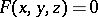in the coordinate system, and its geometric properties are studied by studying its equation by analytic and algebraic means. A curvein space is given as the intersection of two surfacesand. If the respective equations of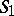andare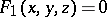and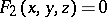, these equations, considered together, are the equation of the curve. Thus, a straight linein space may be considered as the intersection of two planes. In analytic geometry of space a systematic study is made of the so-called algebraic surfaces of the first and second orders. It was found that only planes are algebraic surfaces of the first order. Surfaces of the second order have equations of the type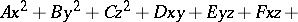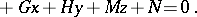The principal method of study and classification of such surfaces is to select a Cartesian coordinate system in which the equation of the surface assumes its simplest form, the latter being then studied. Cf. Surface of the second order.